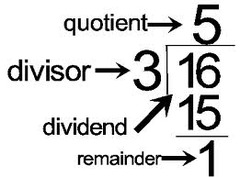Problems
108 - Dividend

Dividend

Time Limit: 1 sec

The Problem

In a division problem, the divisor is D, the quotient is and the remainder is one-third of the divisor. What is the dividend ?

Example:

if D = 3 and Q = 5 then

Dividend is 16The Input

The input file contains only 2 integer numbers D, and (D<=4000, Q<100).

The Output

Output will show the one integer numbers in a separate line.

Sample Input

3 5

Sample Output

16

Problem Setter: Shahin ShamS